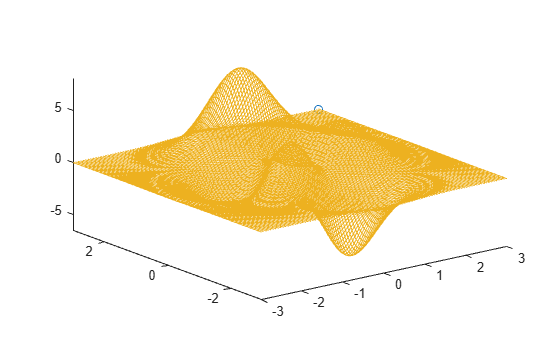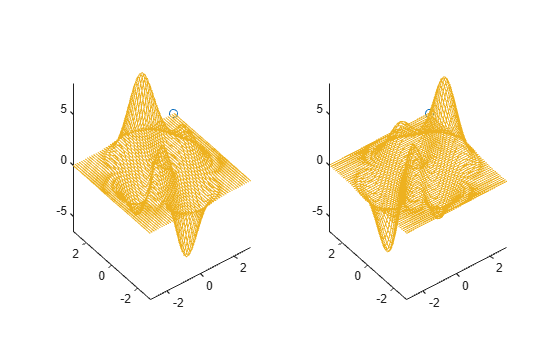# comet3

## 语法

``comet3(z)``
``comet3(x,y,z)``
``comet3(x,y,z,p)``
``comet3(ax,x,y,z,p)``

## 说明

``comet3(z)` 显示 `z` 中指定的数据对与 `z` 的索引相匹配的 `x` 和 `y` 值的彗星图。彗星是由标记（头部）和一条线（尾部）构成的动画，用于跟踪线条随数据点而不断变长的情况。尾巴是跟踪整个函数的实线。`

``comet3(x,y,z)` 显示 `z` 对 `x` 和 `y` 的彗星图。`

``comet3(x,y,z,p)` 指定彗星主体长度。彗星主体是位于头部后的尾部段，此尾部段以不同颜色开始，但会渐变为相同颜色。主体长度为 `p*length(y)`，其中 p 是 [0, 1) 范围内的一个标量。`

``comet3(ax,x,y,z,p)` 将彗星绘制到 `ax` 指定的坐标区中。`

## 示例

```[xmat,ymat,zmat] = peaks(100); xvec = xmat(:); yvec = ymat(:); zvec = zmat(:); comet3(xvec,yvec,zvec)``````[xmat,ymat,zmat] = peaks(100); xvec = xmat(:); yvec = ymat(:); zvec = zmat(:); p = 0.5; comet3(xvec,yvec,zvec,p)``````[xmat,ymat,zmat] = peaks(50); xvec = xmat(:); yvec = ymat(:); zvec = zmat(:); p = 0.25;```

```tiledlayout(1,2); ax1 = nexttile; ax2 = nexttile; comet3(ax1,xvec,yvec,zvec,p) comet3(ax2,yvec,xvec,zvec,p)```## 输入参数

z 值，指定为向量。要绘制对特定 x 和 y 值的图，您还必须指定 `x``y`

x 值，指定为向量。`x``y``z` 的大小必须相同。如果您为 `ax` 指定地理坐标区，则 `x` 表示以度为单位的纬度。

y 值，指定为向量。`x``y``z` 的大小必须相同。如果您为 `ax` 指定地理坐标区，则 `y` 表示以度为单位的经度。

• 彗星图不支持数据提示。